## Physics. Lab 2

Course Description:

This course contains 10 experiments relate to the theoretical course that is taught to students in Physics 101. Students learn how measure physical quantities and analyze the data., in addition to calculate the percentage  error and difference. These experiments focus on mechanics, heat, and fluids, where student perform an experiment every week.

Objectives:

1. To give students an opportunity to apply theories and equations on our life.

2. To train students to measure physical quantities and analyze experimental data.

3.  To understand kinematics in one dimension.

Equipment:

Ruler, Vernier Caliper, Micrometer, Photo-gates, air track, air supplier, pendulum, force table, metal masses, wooden masses, inclined plane, curved track, stop watch, vibrator, stands, thermometer, balance.

Experiments:

1. Uncertainty and errors: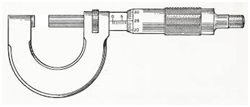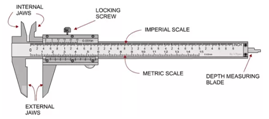2. Force table: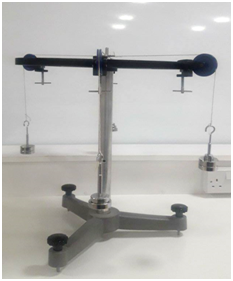3. Motion in one dimension: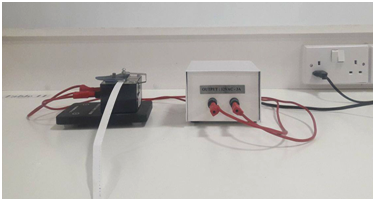4. Projectile motion: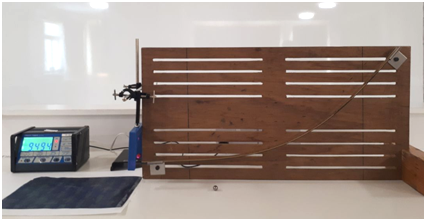5. Newton's second law: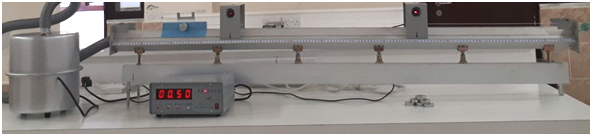6. Friction: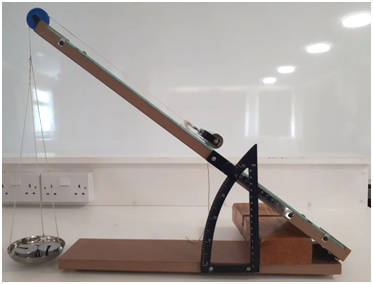7. Collision in two dimension: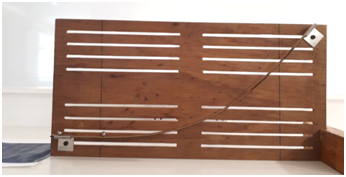8. Simple Harmonic Motion: